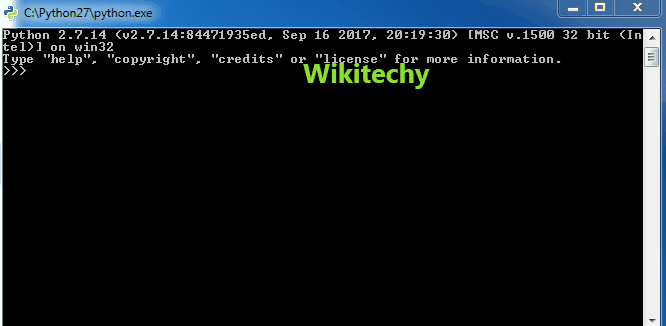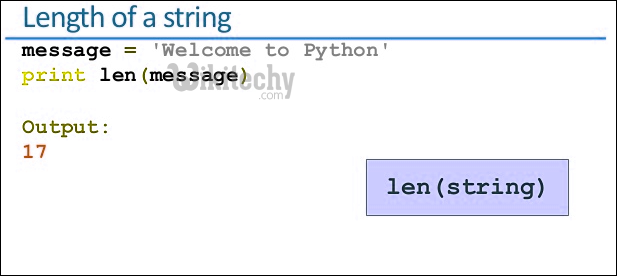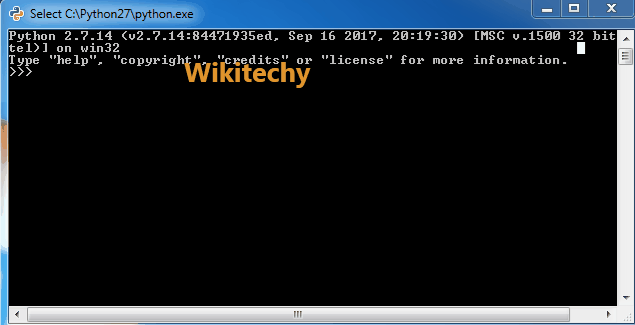# python tutorial - Python String | Python String Methods | Part 2 - len, count, center, ljust, rjust, isalpha, isalnum, isspace & join - learn python - python programmingLearn Python - Python tutorial - python lenth - Python examples - Python programs

1. len() :- This function returns the length of the string.lower cased, otherwise false.

2. count(“string”, beg, end) :- This function counts the occurrence of mentioned substring in whole string. This function takes 3 arguments, substring, beginning position( by default 0) and end position(by default string length).Learn Python - Python tutorial - python str - Python examples - Python programs

python - Sample - python code :

python tutorial - Output :

3. center() :- This function is used to surround the string with a character repeated both sides of string multiple times. By default the character is a space. Takes 2 arguments, length of string and the character.

4. ljust() :- This function returns the original string shifted to left that has a character at its right. It left adjusts the string. By default the character is space. It also takes two arguments, length of string and the character.

5. rjust() :- This function returns the original string shifted to right that has a character at its left. It right adjusts the string. By default the character is space. It also takes two arguments, length of string and the character.

python - Sample - python code :

python tutorial - Output :

6. isalpha() :- This function returns true when all the characters in the string are alphabets else returns false.

7. isalnum() :- This function returns true when all the characters in the string are combination of numbers and/or alphabets else returns false.

8. isspace() :- This function returns true when all the characters in the string are spaces else returns false.

python - Sample - python code :

python tutorial - Output :

9. join() :- This function is used to join a sequence of strings mentioned in its arguments with the string.

python - Sample - python code :Learn Python - Python tutorial - python string - Python examples - Python programs

python tutorial - Output :

Wikitechy tutorial site provides you all the learn python , free python online course , best online course to learn python , learn how to program in python python training free